# 2 》set operation.pdf

30 de May de 2023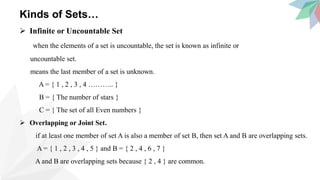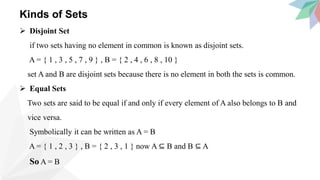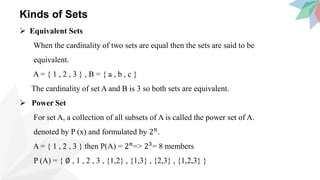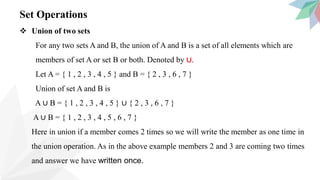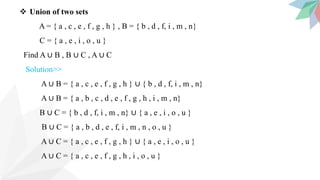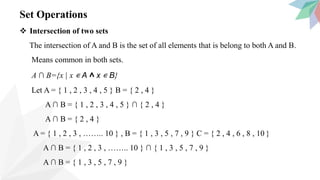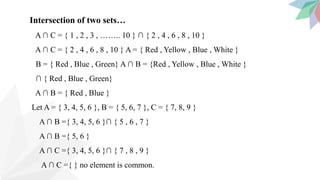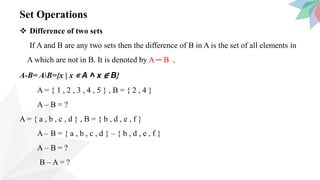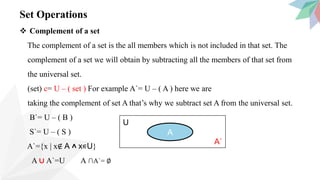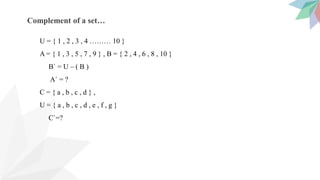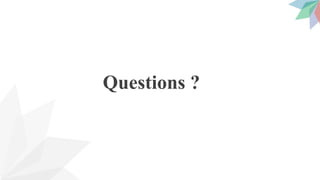1 de 13

### 2 》set operation.pdf

• 1. Set Operations Nabi Safari
• 2. Content  Kind of sets  Set operations
• 3. Kinds of Sets…  Infinite or Uncountable Set when the elements of a set is uncountable, the set is known as infinite or uncountable set. means the last member of a set is unknown. A = { 1 , 2 , 3 , 4 ……….. } B = { The number of stars } C = { The set of all Even numbers }  Overlapping or Joint Set. if at least one member of set A is also a member of set B, then set A and B are overlapping sets. A = { 1 , 2 , 3 , 4 , 5 } and B = { 2 , 4 , 6 , 7 } A and B are overlapping sets because { 2 , 4 } are common.
• 4. Kinds of Sets  Disjoint Set if two sets having no element in common is known as disjoint sets. A = { 1 , 3 , 5 , 7 , 9 } , B = { 2 , 4 , 6 , 8 , 10 } set A and B are disjoint sets because there is no element in both the sets is common.  Equal Sets Two sets are said to be equal if and only if every element of A also belongs to B and vice versa. Symbolically it can be written as A = B A = { 1 , 2 , 3 } , B = { 2 , 3 , 1 } now A ⊆ B and B ⊆ A So A = B
• 5. Kinds of Sets  Equivalent Sets When the cardinality of two sets are equal then the sets are said to be equivalent. A = { 1 , 2 , 3 } , B = { a , b , c } The cardinality of set A and B is 3 so both sets are equivalent.  Power Set For set A, a collection of all subsets of A is called the power set of A. denoted by P (x) and formulated by 2𝑛. A = { 1 , 2 , 3 } then P(A) = 2𝑛 => 23 = 8 members P (A) = { ∅ , 1 , 2 , 3 , {1,2} , {1,3} , {2,3} , {1,2,3} }
• 6. Set Operations  Union of two sets For any two sets A and B, the union of A and B is a set of all elements which are members of set A or set B or both. Denoted by ∪. Let A = { 1 , 2 , 3 , 4 , 5 } and B = { 2 , 3 , 6 , 7 } Union of set A and B is A ∪ B = { 1 , 2 , 3 , 4 , 5 } ∪ { 2 , 3 , 6 , 7 } A ∪ B = { 1 , 2 , 3 , 4 , 5 , 6 , 7 } Here in union if a member comes 2 times so we will write the member as one time in the union operation. As in the above example members 2 and 3 are coming two times and answer we have written once.
• 7.  Union of two sets A = { a , c , e , f , g , h } , B = { b , d , f, i , m , n} C = { a , e , i , o , u } Find A ∪ B , B ∪ C , A ∪ C Solution>> A ∪ B = { a , c , e , f , g , h } ∪ { b , d , f, i , m , n} A ∪ B = { a , b , c , d , e , f , g , h , i , m , n} B ∪ C = { b , d , f, i , m , n} ∪ { a , e , i , o , u } B ∪ C = { a , b , d , e , f, i , m , n , o , u } A ∪ C = { a , c , e , f , g , h } ∪ { a , e , i , o , u } A ∪ C = { a , c , e , f , g , h , i , o , u }
• 8. Set Operations  Intersection of two sets The intersection of A and B is the set of all elements that is belong to both A and B. Means common in both sets. A ∩ B={x | x ∊ A ˄ x ∊ B} Let A = { 1 , 2 , 3 , 4 , 5 } B = { 2 , 4 } A ∩ B = { 1 , 2 , 3 , 4 , 5 } ∩ { 2 , 4 } A ∩ B = { 2 , 4 } A = { 1 , 2 , 3 , …….. 10 } , B = { 1 , 3 , 5 , 7 , 9 } C = { 2 , 4 , 6 , 8 , 10 } A ∩ B = { 1 , 2 , 3 , …….. 10 } ∩ { 1 , 3 , 5 , 7 , 9 } A ∩ B = { 1 , 3 , 5 , 7 , 9 }
• 9. Intersection of two sets… A ∩ C = { 1 , 2 , 3 , …….. 10 } ∩ { 2 , 4 , 6 , 8 , 10 } A ∩ C = { 2 , 4 , 6 , 8 , 10 } A = { Red , Yellow , Blue , White } B = { Red , Blue , Green} A ∩ B = {Red , Yellow , Blue , White } ∩ { Red , Blue , Green} A ∩ B = { Red , Blue } Let A = { 3, 4, 5, 6 }, B = { 5, 6, 7 }, C = { 7, 8, 9 } A ∩ B ={ 3, 4, 5, 6 }∩ { 5 , 6 , 7 } A ∩ B ={ 5, 6 } A ∩ C ={ 3, 4, 5, 6 }∩ { 7 , 8 , 9 } A ∩ C ={ } no element is common.
• 10. Set Operations  Difference of two sets If A and B are any two sets then the difference of B in A is the set of all elements in A which are not in B. It is denoted by A ─ B , A-B= AB={x | x ∊ A ˄ x ∉ B} A = { 1 , 2 , 3 , 4 , 5 } , B = { 2 , 4 } A – B = ? A = { a , b , c , d } , B = { b , d , e , f } A – B = { a , b , c , d } – { b , d , e , f } A – B = ? B – A = ?
• 11. Set Operations  Complement of a set The complement of a set is the all members which is not included in that set. The complement of a set we will obtain by subtracting all the members of that set from the universal set. (set) c= U – ( set ) For example A`= U – ( A ) here we are taking the complement of set A that’s why we subtract set A from the universal set. B`= U – ( B ) S`= U – ( S ) A`={x | x∉ A ˄ x∊U} A ∪ A`=U A ∩A`= ∅ U A` A
• 12. Complement of a set… U = { 1 , 2 , 3 , 4 ……… 10 } A = { 1 , 3 , 5 , 7 , 9 } , B = { 2 , 4 , 6 , 8 , 10 } B` = U – ( B ) A` = ? C = { a , b , c , d } , U = { a , b , c , d , e , f , g } C`=?
• 13. Questions ?